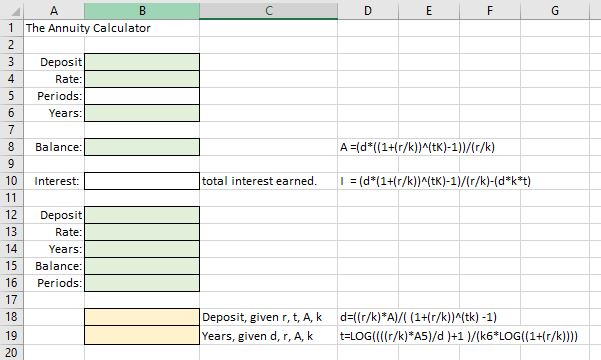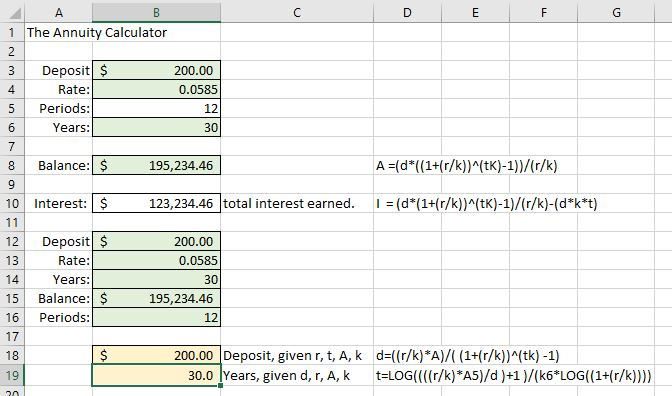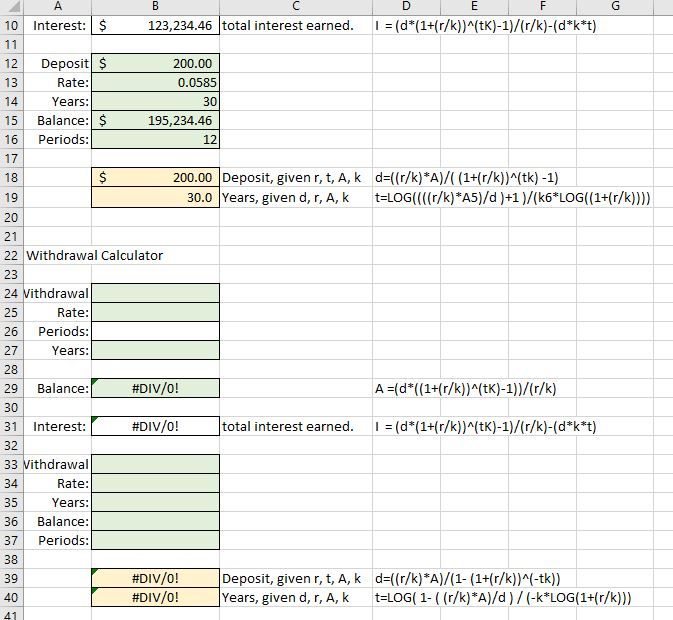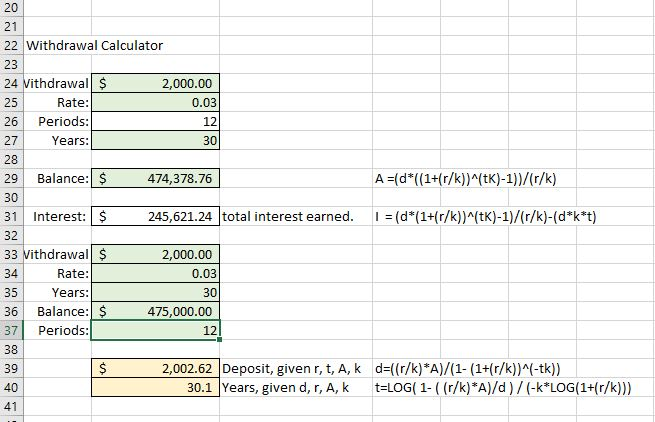## Annuity Calculator: 401(k) Plans, IRAs, and Annuities

### Learning Outcomes

• Calculate annuity balance over time
• Analyze and compare annuities in real-world applications

In the meantime, we will explore the math behind savings annuities and withdrawal annuities by creating spreadsheet calculators for a general long-term savings strategy that simulates a retirement account or annuity.

Recall the annuity formula from earlier in the text.

### Annuity Formula

$P_{N}=\frac{d\left(\left(1+\frac{r}{k}\right)^{Nk}-1\right)}{\left(\frac{r}{k}\right)}$

• PN is the balance in the account after N years.
• d is the regular deposit (the amount you deposit each year, each month, etc.)
• r is the annual interest rate in decimal form.
• k is the number of compounding periods in one year.

If the compounding frequency is not explicitly stated, assume there are the same number of compounds in a year as there are deposits made in a year.

The annuity formula is a tricky one to get right. Doing it by hand requires that you judiciously apply the order of operations. If you try to do it all in one step in your calculator, you’ll have to be super careful of all the parentheses. It can get frustrating! If you get bogged down, just chunk it out into smaller pieces, then put the pieces together at the end. Try to hold the value of decimal approximations as long as you can before rounding to avoid significant rounding errors..

A savings product that is tied to an investment account can earn 3% – 5%, even 6%, yield per year on average over the long run. The key idea is that you leave the money invested as the market rises and drops. Take a bigger risk early in your career, then move your money to more secure holdings at a lower yield as you near retirement.

You deposit $200 per month for 30 years into a savings annuity that yields 5.85% annually, compounded monthly. Use the annuity formula to answer the following. (a) How much will you have in the account after 30 years? (b) How much of the account balance will be interest? ### Spreadsheet Hands-On: Create an Annuity Calculator ### Step 1: Create the savings calculator You can use the compound interest calculator that you made previously to create this one since many of the fields will be similar. Right-click on the Compound Interest tab at the bottom of the sheet to make a copy and set up your fields as shown in the image below. Note that the formulas have been entered in the cells to the right of their respective fields. The Principal label has been changed to Deposit. The total interest earned is now the difference between the final account balance and the deposit times period time number of years invested. This calculator assumes you will deposit regularly each month and make no early withdrawals.Next, we’ll store the formulas. 1. Into cell B8, Balance, type =(B3*((1+(B4/B5))^(B6*B5)-1))/(B4/B5) 2. Into cell B10, Interest, type =B8-(B3*B5*B6) 3. Cells 18 and 19 contain the formula solved for the deposit, and number of years invested, respectively. 4. Into cell B18, type =((B13/B16)*B15)/((1+(B13/B16))^(B14*B16)-1) 5. Into cell B19, type = LOG((((B13/B16)*B15)/B12 )+1 )/(B16*LOG((1+(B13/B16)))) 6. Include the numbers from the example above in the green cells. The Balance, Interest, and the two yellow cells will automatically populate based on your input. When you have finished, it should look like the image below.## Payout Annuities Recall the payout annuity formula from a previous section in the text. It was very similar to the savings annuity formula. ### Payout Annuity Formula $P_{0}=\frac{d\left(1-\left(1+\frac{r}{k}\right)^{-Nk}\right)}{\left(\frac{r}{k}\right)}$ • P0 is the balance in the account at the beginning (starting amount, or principal). • d is the regular withdrawal (the amount you take out each year, each month, etc.) • r is the annual interest rate (in decimal form. Example: 5% = 0.05) • k is the number of compounding periods in one year. • N is the number of years we plan to take withdrawals The payout annuity will calculate the account balance needed to make regular withdrawals over time at a certain rate of interest. Imagine that you’ve spent 30 working years saving your money, building up the annuity by depositing and earning interest, and now you’ll be withdrawing that money as an income stream. Annuities are also used to pay out a settlement or sizable lottery win over time. The account begins with a large amount and the “deposit” under the savings annuity becomes the “withdrawal” under the payout annuity. See the example below for the type of question you might use a calculator to answer. ### Example You are planning for retirement. You know that you’ll be saving at an aggressive rate for the next 30 years, tolerating some risk in your portfolio of investments in exchange for a higher rate of return. You would like to be able to withdraw$2000 every month from your retirement account for a total of 30 years. You know that you won’t tolerate as much risk once you become dependent on the money as income, so you plan for the account to earn 3% interest during retirement. How much will you need in your account when you retire?

Let’s create the withdrawal calculator to help us answer this and other questions about payout annuities such as retirement accounts.

### Step 2: Create the withdrawal calculator

1. To create the withdrawal portion of the spreadsheet, copy the savings calculator from fields A1 – D19 and paste them into the same spreadsheet. Place your cursor into cell A22 then choose paste (or CTL-V). Change the description “The Annuity Calculator” to “Withdrawal Calculator.”
2. You can leave the formula cells populated with their formulas, but empty the others. It should look like the image below when you’ve emptied them. Note that the template formulas next to the formula cells have already been changed to the withdrawal formula.3. Into cell B29, type =(B24*(1-(1+(B25/B26))^(-B27*B26)))/(B25/B26)
4. Into cell B31, type =(B24*B26*B27)-B29
5. Into cell B39, type =((B34/B37)*B36)/(1-(1+(B34/B37))^(-B35*B37))
6. Into cell B40, type =LOG(1-((B34/B37)*B36)/B33)/(-B37*LOG(1+(B34/B37)))
7. Populate the shaded cells with the information from the example above to test that your formulas work. The yellow shaded areas should auto-populate as you fill in cells in row 33 – 37. When you are done your spreadsheet should look like the image below.Note that the amount of money you’ll actually withdraw from the account over 30 years is $720,000, much more than the account balance you’ll need. The difference between the balance and your withdrawals is the interest earned by continuing to invest the funds while making withdrawals. This is the real power of long-term savings. ### Step 3: Use the annuity calculator Use your calculator to answer the questions in the following examples of real-world situations. ### example You have decided to open an annuity to save$300 each month. You find one that will earn 2% interest, compounded monthly.

(a) How much will you have in the account if you leave your money in for 15 years?

(b) How much money will you have deposited into the account after 15 years?

(c) How much interest will you have earned after 15 years of monthly $300 deposits? (d) How much money would you need to deposit monthly to earn$55,000 total?

(e) How many years would you need to continue depositing $300 into the account to build a balance of$150,000?

### example

You have saved $400,000 for retirement and will begin taking withdrawals from your annuity that pays 4% interest, compounded monthly. (a) What monthly withdrawal can you make if you want to keep withdrawing for 20 years? (b) What monthly withdrawal can you make if you want to keep withdrawing for 30 years? (c) If you take out$3000 a month, how many years will the money last?